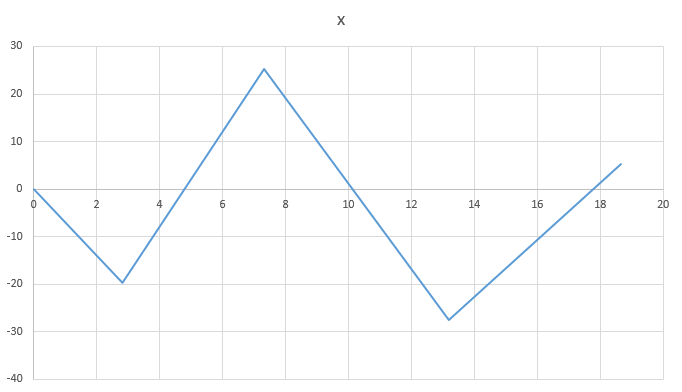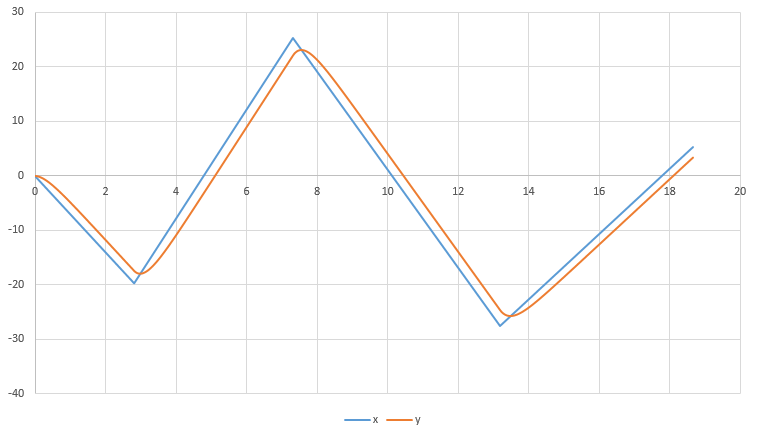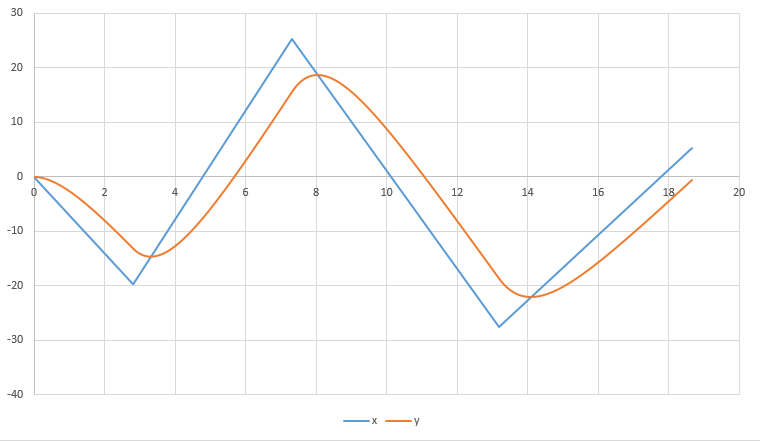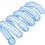# Generating Smooth Curves Using Simple Digital Filters

Suppose we have a function of a variable $t$, $x(t)$, which consists of multiple connected line segments.What if we wanted to make a version of this curve with the jagged edges smoothed out? This is fairly easy to do using a digital filter. Define the smoothed function, $y$, in the following way.

$\large{y_k = \alpha y_{k-1} + (1-\alpha) x_k}$

If we discretize $t$, the $k$ subscript denotes the present iteration, and the $k-1$ subscript denotes the previous iteration. The equation above is known as an "infinite impulse response" (IIR) filter, because the present filter output is a function of the previous output, and so on, and so on.....The filter will take infinitely many iterations to fully catch up to any step change in the input.

The present value of $y$ ( the smoothed version of $x$), is equal to a weighted sum of the previous value of $y$ and the present value of $x$. Thus, by tweaking the value of the "inertia constant" $\alpha$, we can dictate how fast the value of $y$ can change, and thus, how much smoothing we get.

As an extreme case, suppose $\alpha = 1$. This would mean that $y$ would be frozen at a static value forever (infinite inertia). For $\alpha = 0$, the smoothed and un-smoothed curves are exactly equal, corresponding to zero inertia.

Some graphs for intermediate values of $\alpha$ are shown below. The third one is particularly reminiscent of the Fourier series. We could create a saw-tooth wave by summing up sine waves with various frequencies (from low to high). By increasing the inertia, we essentially implement a low-pass filter which removes the higher harmonics from the saw-tooth, revealing lower-frequency sinusoids.

$\alpha = 0.97$$\alpha = 0.99$$\alpha = 0.998$Note by Steven Chase
4 years, 1 month ago

This discussion board is a place to discuss our Daily Challenges and the math and science related to those challenges. Explanations are more than just a solution — they should explain the steps and thinking strategies that you used to obtain the solution. Comments should further the discussion of math and science.

When posting on Brilliant:

• Use the emojis to react to an explanation, whether you're congratulating a job well done , or just really confused .
• Ask specific questions about the challenge or the steps in somebody's explanation. Well-posed questions can add a lot to the discussion, but posting "I don't understand!" doesn't help anyone.
• Try to contribute something new to the discussion, whether it is an extension, generalization or other idea related to the challenge.
• Stay on topic — we're all here to learn more about math and science, not to hear about your favorite get-rich-quick scheme or current world events.

MarkdownAppears as
*italics* or _italics_ italics
**bold** or __bold__ bold
- bulleted- list
• bulleted
• list
1. numbered2. list
1. numbered
2. list
Note: you must add a full line of space before and after lists for them to show up correctly
paragraph 1paragraph 2

paragraph 1

paragraph 2

[example link](https://brilliant.org)example link
> This is a quote
This is a quote
    # I indented these lines
# 4 spaces, and now they show
# up as a code block.

print "hello world"
# I indented these lines
# 4 spaces, and now they show
# up as a code block.

print "hello world"
MathAppears as
Remember to wrap math in $$ ... $$ or $ ... $ to ensure proper formatting.
2 \times 3 $2 \times 3$
2^{34} $2^{34}$
a_{i-1} $a_{i-1}$
\frac{2}{3} $\frac{2}{3}$
\sqrt{2} $\sqrt{2}$
\sum_{i=1}^3 $\sum_{i=1}^3$
\sin \theta $\sin \theta$
\boxed{123} $\boxed{123}$

## Comments

There are no comments in this discussion.

×

Problem Loading...

Note Loading...

Set Loading...